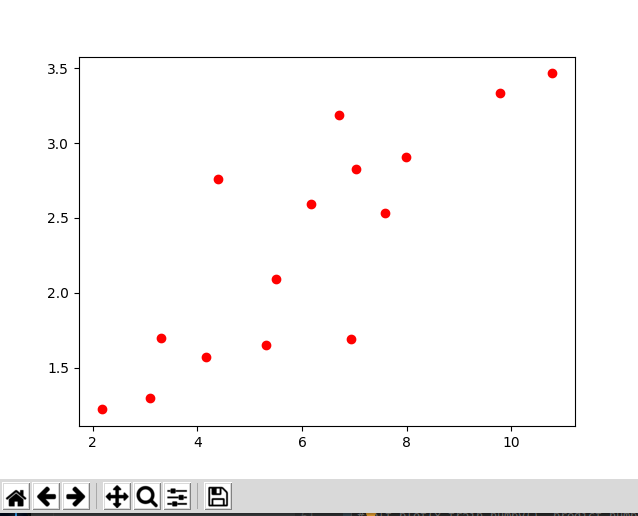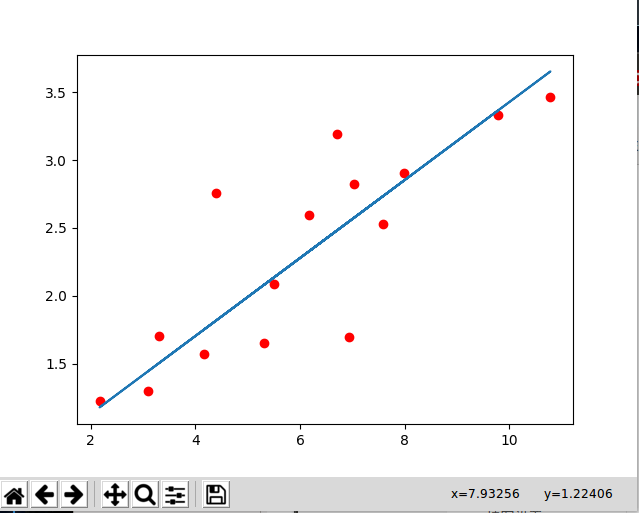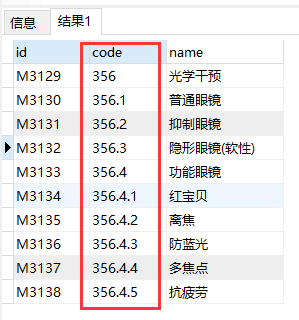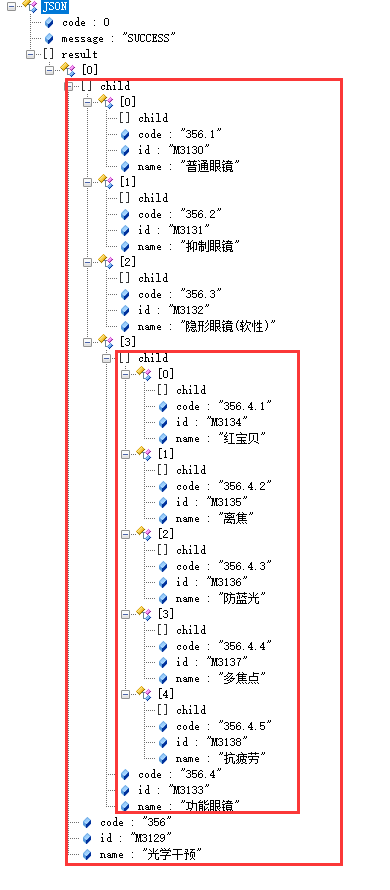• 在本示例教程中将会按照下面的规范优化线形条码的输出： Barcode width = 60 mm Barcode height = 30 mm Resolution of the output device = 200 dpi (dots per inch) 在此规范基础上，我们首先需要根据目标设备...
概述：
在本示例教程中将会按照下面的规范优化线形条码的输出：
Barcode width = 60 mm
Barcode height = 30 mm
Resolution of the output device = 200 dpi (dots per inch)
在此规范基础上，我们首先需要根据目标设备像素计算条码的尺寸。接下来对这个尺寸(实际上只有宽度)加以调整，以便条码的每个条和每个间隙都完全匹配输出光栅。高度调整不是必需的，因为扫描过程通常是不受条形码高度的影响。
首先我们计算条码宽度：
因此需要把宽度(默认单位mm)转换成英寸为单位。然后根据输出设备的分辨率(dots per inch)计算结果。
60 / 25.4 * 200 @ 472.44 dots (or pixels)
》》》免费下载TBarCode SDK最新版
然后我们计算出模块宽度并采用.............》》》查看原文

展开全文SDK TBarCode
• 基于MATLAB语言的拱桥拱肋线形优化设计.pdf
• 传送门 题解：思考一下可以知道在一个区间内连续进行+/−+/-+/−操作后，这个区间的xxx的变化是连续的，也就是找到变化中的最大值和最小值即可，最后也就是维护下区间最大最小值而已，对于中间跳过一段操作，对于...
传送门
题解：思考一下可以知道在一个区间内连续进行$+/-$操作后，这个区间的$x$的变化是连续的，也就是找到变化中的最大值和最小值即可，最后也就是维护下区间最大最小值而已，对于中间跳过一段操作，对于后面的影响无非就是减去这段对于$x$的影响罢了。
#include <bits/stdc++.h>
using namespace std;
const int N = 2e5 + 5;
int n, m, x, y, a[N];
string s;
struct node{
int l, r, mx, mn;
};
node tree[N << 2];
void build(int p, int l, int r)
{
tree[p].l = l; tree[p].r = r;
if (l == r) {
tree[p].mx = tree[p].mn = a[l];
return ;
}
int mid = (l + r) >> 1;
build(p << 1, l, mid);
build(p << 1 | 1, mid + 1, r);
tree[p].mx = max(tree[p << 1].mx, tree[p << 1 | 1].mx);
tree[p].mn = min(tree[p << 1].mn, tree[p << 1 | 1].mn);
}
pair<int, int> ask(int p, int l, int r)
{
if (tree[p].l >= l && tree[p].r <= r) {
return {tree[p].mx, tree[p].mn};
}
int mid = (tree[p].l + tree[p].r) >> 1;
if (r <= mid) {
return ask(p << 1, l, r);
} else if (l > mid) {
return ask(p << 1 | 1, l, r);
} else {
auto tl = ask(p << 1, l, mid), tr = ask(p << 1 | 1, mid + 1, r);
return {max(tl.first, tr.first), min(tl.second, tr.second)};
}
}
int main()
{
int T;
cin >> T;
while (T--) {
cin >> n >> m >> s;
for (int i = 0; i < n; i++) {
a[i+2] = a[i+1] + (s[i] == '+' ? 1 : -1);
}
n += 2; a[n] = a[n - 1];
build(1, 1, n);
for (int i = 0; i < m; i++) {
cin >> x >> y;
x += 1; y += 1;
int t = a[y] - a[x - 1];
auto l = ask(1, 1, x - 1), r = ask(1, y, n);
r.first -= t; r.second -= t;
cout << max(l.first, r.first) - min(l.second, r.second) + 1 << endl;
}
}
return 0;
}


如上我的做法为$O(nlogn)$级别，官方题解为$O(n)$级别
思想还是那样的思想，但是维护就得思考一番，考虑用$pr[i]$表示当前到了第$i$位时的数字是多少，$prl[i]、prr[i]$为前$i$长度的最小的，最大的，维护非常简单
$prl[i]=min(prl[i-1],pr[i]+d)\\ prr[i]=max(prr[i-1],pr[i]+d)$
但是后缀就有点技巧了，考虑这样一件事情，如果已经知道$sul[i]$表示从第$i$位开始的最小的，那么第$i-1$位呢，肯定是$sul[i-1]=sul[i]+d$，如果为正数也就是$1$，那么改为$0$即可，如果不是这个式子，那换成其他的只能更大，不可能最小，同理最大也是一样的。
最后维护答案时直接维护$pr[l-1]$的值即可
#include <bits/stdc++.h>
using namespace std;
int n, m, l, r;
string s;
int main()
{
int T;
cin >> T;
while (T--) {
cin >> n >> m >>s;
vector<int> sul(1, 0), sur(1, 0), prl(1, 0), prr(1, 0), pr(1, 0);
for (int i = n - 1; i >= 0; i--) {
int d = s[i] == '+' ? 1 : -1;
sul.emplace_back(min(0, sul.back() + d));
sur.emplace_back(max(0, sur.back() + d));
}
reverse(sul.begin(), sul.end());
reverse(sur.begin(), sur.end());
for (int i = 0; i < n; i++) {
int d = s[i] == '+' ? 1 : -1;
pr.emplace_back(pr.back() + d);
prl.emplace_back(min(prl.back(), pr.back()));
prr.emplace_back(max(prr.back(), pr.back()));
}
for (int i = 0; i < m; i++) {
cin >> l >> r;
l--;
int l1 = prl[l], r1 = prr[l];
int l2 = sul[r] + pr[l], r2 = sur[r] + pr[l];
cout << max(r1, r2) - min(l1, l2) + 1 << endl;
}
}
return 0;
}




展开全文• 今天做的是一个用pytorch训练出来的一元线形回归模型；如图，我们先找一些样本点，用 matplotlib.pyplot显示如上，我们把它加载到内存里面，然后通过pytorch的模组简单自定义一下前向函数就可以了，完整代码如下 ...今天做的是一个用pytorch训练出来的一元线形回归模型；如图，我们先找一些样本点，用

matplotlib.pyplot显示如上，我们把它加载到内存里面，然后通过pytorch的模组简单自定义一下前向函数就可以了，完整代码如下

#!/usr/bin/python
# -*- coding: UTF-8 -*-

import torch
import torch.nn.functional as F
import matplotlib.pyplot as plt
import numpy as np

x_train = np.array([[3.3], [4.4], [5.5], [6.71], [6.93], [4.168],
[9.779], [6.182], [7.59], [2.167], [7.042],
[10.791], [5.313], [7.997], [3.1]], dtype=np.float32)

y_train = np.array([[1.7], [2.76], [2.09], [3.19], [1.694], [1.573],
[3.336], [2.596], [2.53], [1.221], [2.827],
[3.465], [1.65], [2.904], [1.3]], dtype=np.float32)
//将数据从numpy转为tensor,pytorch中数据都是以tensor的形式处理
x_train = torch.from_numpy(x_train)
y_train = torch.from_numpy(y_train)
//通过pytorch的内置模组自实现一个一元线形回归
class LinearRegression(torch.nn.Module):
def __init__(self):
super(LinearRegression,self).__init__() //固定格式
self.linear = torch.nn.Linear(1, 1) //输入和输出都是一维的

def forward(self, x): //前向传递方法
out = self.linear(x)
return out
//如果有GUP支持的话，将网络放在GPU上
if  torch.cuda.is_available():
model = LinearRegression().cuda()
else:
model = LinearRegression()
//定义损失函数和优化器
criterion = torch.nn.MSELoss()
optimizer = torch.optim.SGD(model.parameters(), lr=1e-3)

num_epochs = 1000
for epoch in range(num_epochs):
if torch.cuda.is_available():
inputs = Variable(x_train).cuda()
target = Variable(y_train).cuda()
else:
inputs = Variable(x_train)
target = Variable(y_train)

out = model(inputs)    //计算出前向传递结果
loss = criterion(out, target) //计算出和真实值的误差

loss.backward() //返回传播
optimizer.step() //优化网络里面的参数

if(epoch + 1) % 20 == 0:
print('Epoch[{}/{}], loss: {:.6f}'.format(epoch + 1, num_epochs, loss.item()))

model.eval() //转为测试模式
predict = model(Variable(x_train).cuda())
predict = predict.data.cpu()
plt.plot(x_train.numpy(), y_train.numpy(), 'ro', label='Original data')
plt.plot(x_train.numpy(), predict.numpy(), label='Fitting data')
plt.show()

运行以上代码，可以得到如下的一个线形函数展开全文pytorch
• 数据库中数据结构图 封装数据的实体类 @Data public class MicroCodeDto { private String id; private String code; private String name; private List<MicroCodeDto>... ...
数据库中数据结构图封装数据的实体类
@Data
public class MicroCodeDto {
private String id;
private String code;
private String name;
private List<MicroCodeDto> child = new ArrayList<>();

public MicroCodeDto(String id, String code, String name) {
this.id = id;
this.code = code;
this.name = name;
}
}

解析方法
public static Object formatXDMCheckOptions(List<MicroCodeDto> options) {
ArrayList<MicroCodeDto> result = new ArrayList<>();
// 使用map保存所有的字段
HashMap<String, MicroCodeDto> map = new HashMap<>();
options.forEach(bean -> map.put(bean.getCode(), bean));
// 查找并填充父子菜单
options.forEach(bean -> {
// 获取最后一个'.'的index
int index = bean.getCode().lastIndexOf(".");
// 没有'.'说明是顶级菜单
if (index == -1) {
} else if (index + 1 < bean.getCode().length()) {
// 子菜单 用最后一个点 分割 找到父菜单code
String newStr = bean.getCode().substring(0, index);
// 找到父菜单
MicroCodeDto fatherDto = map.get(newStr);
// 将所有子菜单 填充到父菜单中
if (!fatherDto.getChild().contains(bean)) {
}
}
});
return result;
}

返回结果展示展开全文• 回归是基于已有的数据对新的数据进行预测，除了标准的线形回归，还会有戴明回归、lasso回归、岭回归、弹性网络回归、逻辑回归等，具体的定义和差别会在下文实现中体现。 　tensorflow作为流行的机器学习框架，有...
• 线形规划最优问题，是指目标函数和约束条件都是线形的函数，所以叫线形规划，我们需要的就是求出在约束条件下最优的目标函数。 二.matlab求解 求解指令为[x,fval]=linprog(f,A,b,Aeq,beq,lb,ub); f:价值向量 A：耗费...
• 将非线形互补问题转化为约束的优化问题，在已经的利用内点障碍函数方法求解约束优化问题的基础上，提出了利用障碍函数方法求解非线形互补问题的采用序列无约束最小化方法(SUMT)的算法，并利用障碍函数的单调性证明了...
• 针对目前抛物线形断面最优水力参数及抛物线方程指数计算尚没有比较... 精度分析及算例计算结果表明:最优水力参数的提出将为该类断面的进一步优化设计提供可靠依据;最优抛物线形断面比其他水力最优断面经济指标更好。
• 考虑弯矩对加劲梁轴向刚度的影响，在迭代计算过程中，提出采用非线性规划方法，并与传统的影响矩阵法进行对比，计算结果表明，两者结果吻合，从而使借助大型通用优化软件对自锚式悬索桥主缆线形进行精确求解成为可能...
• 提出了一种基于线形腔光纤激光器结构的光学反馈系统，对系统输出功率变化表达式进行...通过适当调节反馈腔腔长与目标靶反射率可优化系统反馈信号的灵敏度.根据以上特性，该系统可应用于光学测量领域，例如位移、距离、
• 假设 特征 和 结果 都满足线性。即不大于一次方。这个是针对 收集的数据而言。 收集的数据中，每一个分量，就可以看做一个特征...这个算法只是最优化原理中的一个普通的方法，可以结合最优化原理来学，就容易理解了。
• 本节主要学习线性优化问题。线性优化是最简单的一种优化问题。在我们系统地学习凸优化之前，先回顾下线性优化的知识。虽然最优化可以追溯到十分古老的极值问题，但是它成为一门独立的学科是在本世纪40年代末，是在...线性规划
• 采用一阶最优化计算方法，以成桥后结构的弯曲应变能和主粱的线形为目标函数，以斜拉索的初始张拉力和主梁节段的预抛高值为设计变量，建立了斜拉桥施工控制的空间非线性有限元分析模型，并以某大跨度预应力混凝土斜拉...
• 同理3~5的石子总和我们可以用1~5的石子总和减去1~2的石子总和来求： sum-sum 优化我们的循环框架： for(int i=n-1;i>=1;i--) for(int j=i+1;j;j++) for(int k=i;k;k++) f[i][j] = min(f[i][j], f[i][k]...动态规划 算法 java
• 下面继续添加代码优化显示效果，注意下面的代码要放到 chart.write(document.getElementById("chartContainer")); 上面执行。 var categoryAxis = chart.categoryAxis; categoryAxis.autoGridCount = ...
• Android app 性能优化之视图优化 前言： 每当一款App开始快速扩展的时候，随着业务功能的越来越复杂，功能模块的越来越多总会引起这样那样的性能问题。交互不流畅卡顿，ANR，手机发热量大等等性能问题在Android...Android app 程序员
• 文章目录三、简易线形图（一）调整图形：线条的颜色与风格（二）调整图形：坐标轴上下限（三）设置图形标签 三、简易线形图 所有图形中，最简单的应该就是线性方程 y=f(x)y = f(x)y=f(x) 的可视化了。 要画...python 数据可视化
• 滑动优化 上面的第五步，当手指抬起的时候视图继续滑动一段距离。这种fling效果是通过一个子线程动态减少滑动的值然后更新视图实现的。效果虽然实现了，但是不够流畅，其实安卓已经提供了速度相关的api供我们使用...canvas 控件 android
• 为了改善激光热处理硬化层分布的均匀性，在分析国内外激光热处理光束优化系统研究现状及存在问题的基础上，提出了光束优化系统的两种方案，激光扫描环形光斑和线形光斑。建立了两种光斑温度场的数学模型，模拟了温度...
•  (1)什么是非线性优化问题。(2)什么是非线性凸优化问题。...最优化问题在现实生活中许多重要的问题，都涉及到选区一个最好的目标，或者为达到这个目标而选择某些参数、确定某些值，这些问题都可以归结为最优化问题。算法 excel
• 2线形图 3''' 4# matplotlib 数据可视化库 5 6import matplotlib.pyplot as plt 7 8# pandas 数据提炼库 9 10import pandas as pd 基本线性图 1''' 2基本线性图 3''' 4import numpy as np 5python 数据可视化
• 通过 profile clock工具找出效率很低的部分，使用线形汇编继续优化。线性汇编语言是 C6000系列 DSP独有的一种编程语言，介于高级语言和低级语言之间。
• ## 最优化问题-线性优化(LP)

万次阅读 多人点赞 2017-07-01 19:10:11
这里最优化问题的讨论，主要指在给定某个确认的目标函数以及该函数的自变量的一些约束条件，求函数的最大或最小值的问题，通用的数学表达式: 目标函数 : f(x) f(x) 约束条件 : s.t.g(x)≤0,h(x)=0 s.t. g(x) \leq...algorithm...